# Samacheer Kalvi 7th Maths Solutions Term 1 Chapter 4 Direct and Inverse Proportion Ex 4.1

Students can Download Maths Chapter 4 Direct and Inverse Proportion Ex 4.1 Questions and Answers, Notes Pdf, Samacheer Kalvi 7th Maths Book Solutions Guide Pdf helps you to revise the complete Tamilnadu State Board New Syllabus and score more marks in your examinations.

## Tamilnadu Samacheer Kalvi 7th Maths Solutions Term 1 Chapter 4 Direct and Inverse Proportion Ex 4.1

Fill in the blanks.

(i) If the cost of 8 apples is 56 then the cost of 12 apples is ____.
(ii) If the weight of one fruit box is $$3 \frac{1}{2}$$ kg, then the weight of 6 such boxes is ____.
(iii) A car travels 60 km with 3 liters of petrol. If the car has to cover the distance of
200 km, it requires ___ liters of the petrol.
(iv) If 7 m cloth costs ₹ 294, then the cost of 5m of cloth is ____.
(v) If a machine in a cool drinks factory fills 600 bottles in 5 hrs, then it will fill _____ bottles in 3 hours.
Solutions:
(i) 84
(ii) 21 kg
(iii) 10
(iv) ₹ 210
(v) 360

Question 2.
Say True or False
(i) Distance travelled by a bus and time taken are in direct proportion.
(ii) Expenditure of a family to number of members of the family are in direct proportion.
(iii) Number of students in a hostel and consumption of food are not in direct proportion.
(iv) If Mallika walks 1km in 20 minutes, then she can convert 3km in 1 hour.
(v) If 12 men can dig a pond in 8 days, then 18 men can dig it in 6 days.
Solutions:
(i) True
(ii) True
(iii) False
(iv) True
(v) FalseQuestion 3.
A dozen bananas costs ₹ 20. What is the price of 48 bananas ?
Solution:
Let the required price be ₹ x. As the number of bananas increases price also increases
∴ Number of bananas and cost are in direct proportion.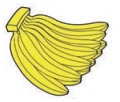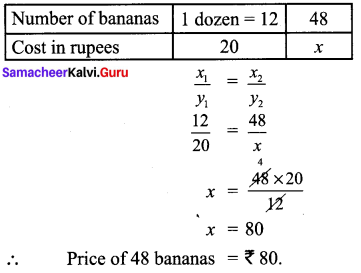Question 4.
A group of 21 students paid ₹ 840 as the entry fee for a magic show. How many students entered the magic show if the total amount paid was ₹ 1680?
Solution:
Let the required number of students be x.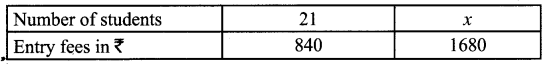As the number of students increases the entry fees also increases.
∴ They are in direct proportion .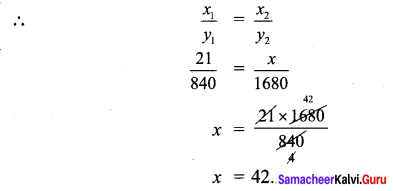∴ The number of students entered magic show = 42Question 5.
A birthday party is arranged in third floor of a hotel. 120 people take 8 trips in a lift to go to the party hall. If 12 trips were made how many people would have attended the party?
Solution:
Let the number of people attended the party be x.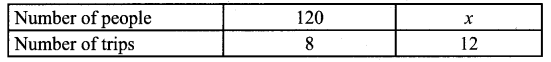As the number of trips increases, number of people also increases.
∴ They are in direct proportion.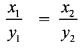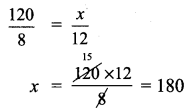180 people attend the party in 12 trips

Question 6.
The shadow of a pole with height of 8m is 6m. If the shadow of another pole measured at the same time is 30m, find the height of the pole?
Solution:
Let the required height of the pole be ‘x’ m.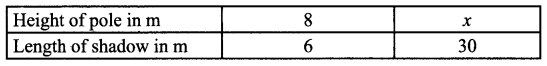Height of the pole and its shadow are in direct proportion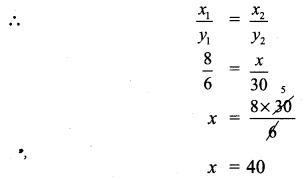∴ Height of the pole x = 40m.Question 7.
A postman can sort out 738 letters in 6 hours. How many letters can be sorted in 9 hours?
Solution:
Let the required number of letters be x.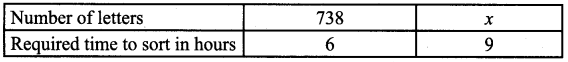They are in direct proportion.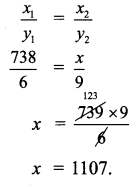In 9 hours 1107 letters can be sorted.

Question 8.
If half a meter of cloth costs ₹ 15. Find the cost of $$8 \frac{1}{3}$$ meters of the same cloth.
Solution:
Let the cost of cloth required be x.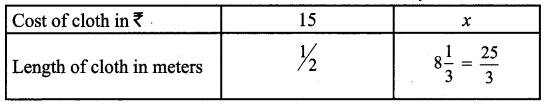Cost and length are in direct proportion.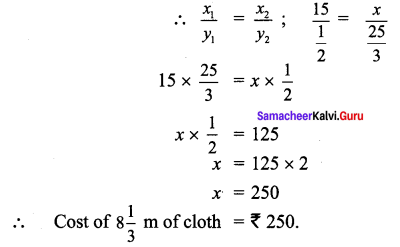Question 9.
The weight of 72 books is 9 kg. What is the weight of 40 such books (using unitary method)
Solution:
Weight of 72 books = 9 kg = 9000 g
∴ Weight of 1 book = $$\frac{9000}{72}$$ = 125 g
∴ Weight of 40 books = 125 × 40 g = 5000 g = 5 kg.
Weight of 40 books = 5 kgQuestion 10.
Thamarai pages ₹ 7500 as rent for 3 months. With the same rate how much does she have to pay for 1 year (using unitary method).
Solution:
Rent paid by Thamarai for 3 months = ₹ 7500
∴ Rent paid for 1 month = $$\frac{7500}{3}$$ = 2500
Rent paid for 1 year or 12 moths = 2500 × 12 = ₹ 30,000
For 1 year rent to be paid = ₹ 30,000

Question 11.
If 30 men can reap a field in 15 days, then in how many days can 20 men reap the same field? (using unitary method).
Solution: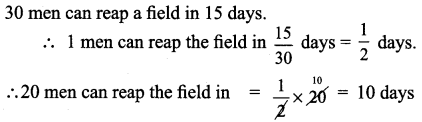∴ 20 men can reap the field in 10 days.

Question 12.
Valli purchase 10 pens for ₹ 180 and Kamala boys 8 pens for ₹ 96. Can you say who bought the pen cheaper (using unitary method).
Solution: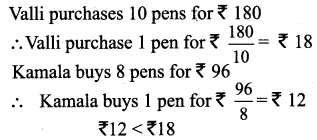∴ Kamala bought the pen cheaper.Question 13.
A motorbike requires 2 liters of petrol to cover 100 kilometres. How many liters of petrol will be required to cover 250 kilometers? (using unitary method).
Solution:
To cover 100 km quantity of petrol required = 2 litres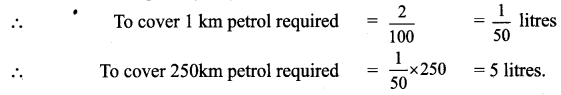5 litres of petrol required to cover 250 km

Objective Type Questions

Question 14.
If the cost of 3 books is ₹ 90, then find the cost of 12 books.
(i) ₹ 300
(ii) ₹ 320
(iii) ₹ 360
(iv) ₹ 400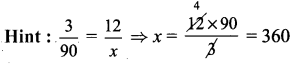Solution:
(iii) ₹ 360Question 15.
If Mani buys 5 kg of potatoes for ₹ 75 then he can buy ₹ 105.
(i) 6
(ii) 7
(iii) 8
(iv) 5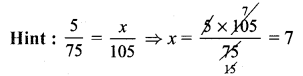Solution:
(ii) 7

Question 16.
35 cycles were produced in 5 days by a company then ___ cycles will be produced in 21 days.
(i) 150
(ii) 70
(iii) 100
(iv) 147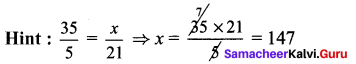Solution:
(iv) 147

Question 17.
An aircraft can accommodate 280 people in 2 trips. It can take ______ trips to take 1400 people.
(i) 8
(ii) 10
(iii) 9
(iv) 12
Solution:
(ii) 10

Question 18.
Suppose 3 kg of sugar is used to prepare sweets for 50 members, then ___ kg of sugar is required for 150 members.
(i) 9
(ii) 10
(iii) 15
(iv) 6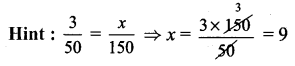Solution:
(i) 9